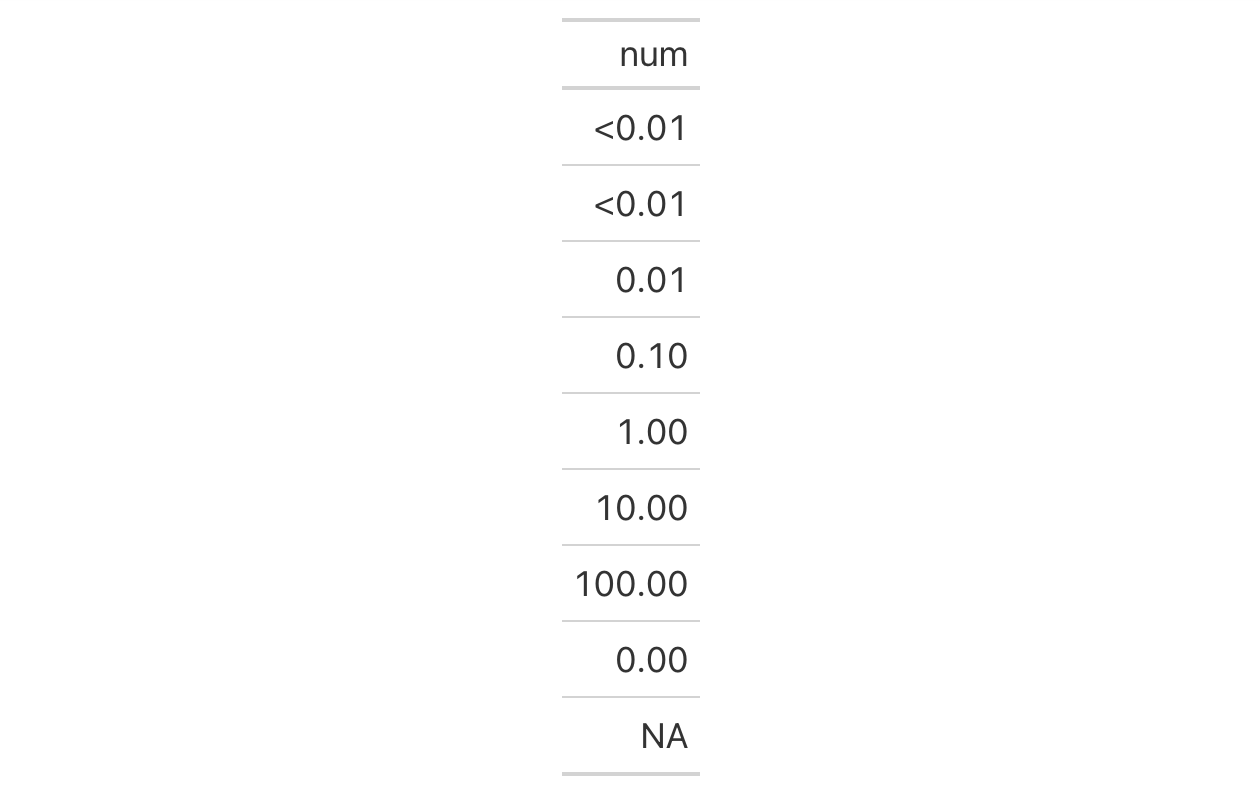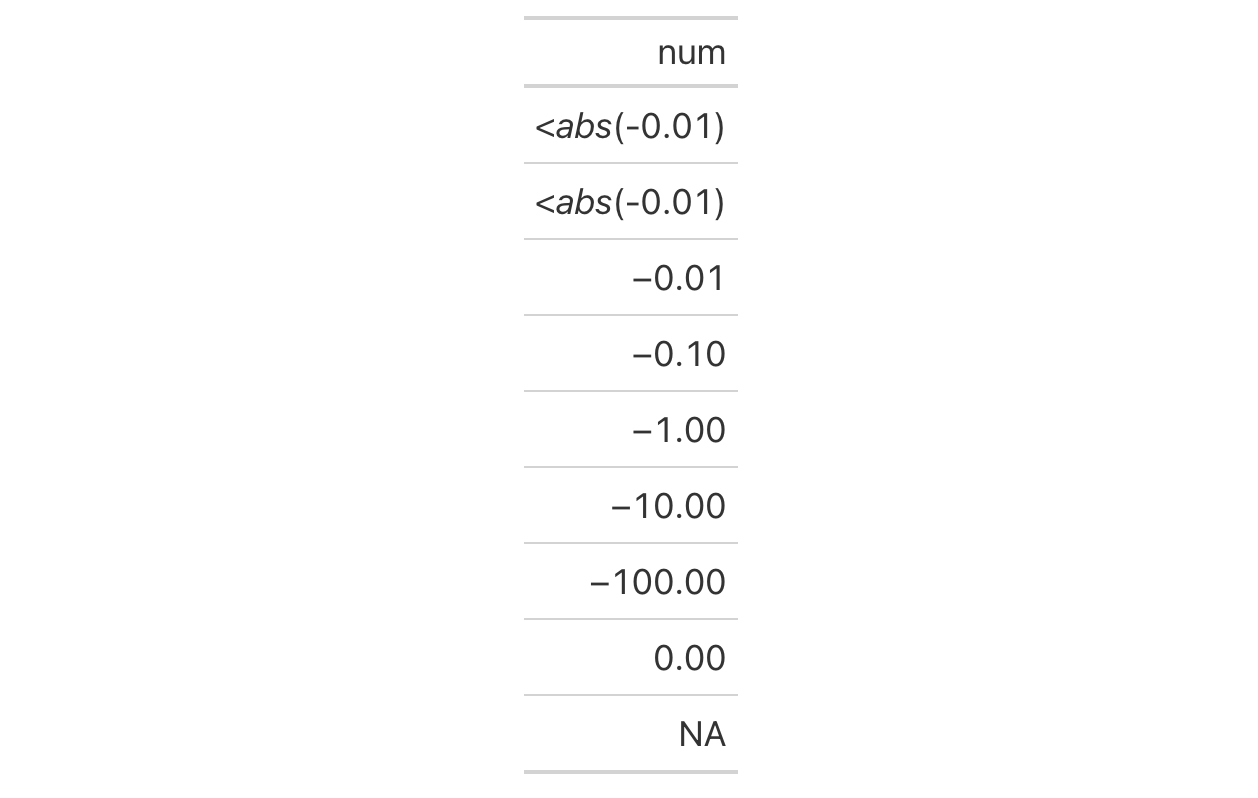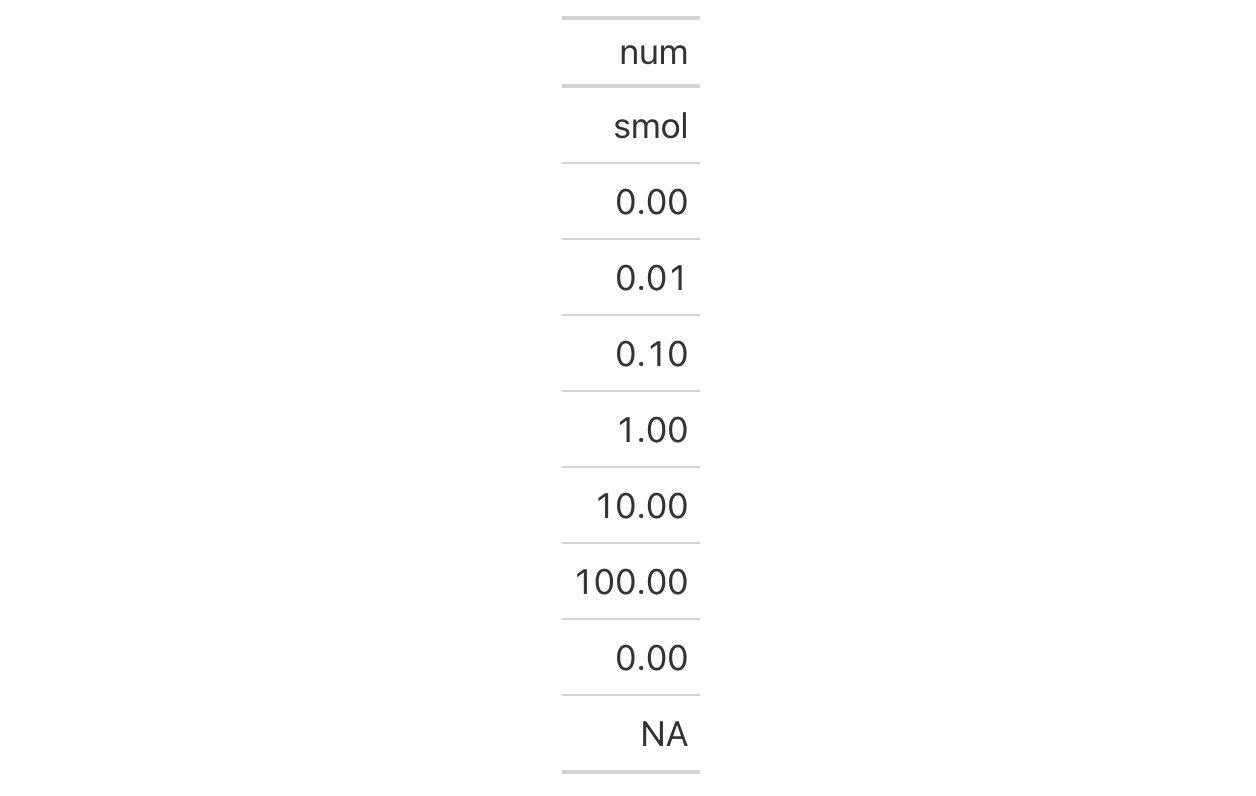Wherever there is numerical data that are very small in value, replacement text may be better for explanatory purposes. The sub_small_vals() function allows for this replacement through specification of a threshold, a small_pattern, and the sign of the values to be considered.

## Usage

sub_small_vals(
data,
columns = everything(),
rows = everything(),
threshold = 0.01,
small_pattern = if (sign == "+") "<{x}" else md("<*abs*(-{x})"),
sign = "+"
)

## Arguments

data

A table object that is created using the gt() function.

columns

The columns to format. Can either be a series of column names provided in c(), a vector of column indices, or a helper function focused on selections. The select helper functions are: starts_with(), ends_with(), contains(), matches(), one_of(), num_range(), and everything().

rows

Optional rows to format. Providing everything() (the default) results in all rows in columns being formatted. Alternatively, we can supply a vector of row captions within c(), a vector of row indices, or a helper function focused on selections. The select helper functions are: starts_with(), ends_with(), contains(), matches(), one_of(), num_range(), and everything(). We can also use expressions to filter down to the rows we need (e.g., [colname_1] > 100 & [colname_2] < 50).

threshold

The threshold value with which values should be considered small enough for replacement.

small_pattern

The pattern text to be used in place of the suitably small values in the rendered table.

sign

The sign of the numbers to be considered in the replacement. By default, we only consider positive values ("+"). The other option ("-") can be used to consider only negative values.

## Value

An object of class gt_tbl.

## Details

Targeting of values is done through columns and additionally by rows (if nothing is provided for rows then entire columns are selected). Conditional formatting is possible by providing a conditional expression to the rows argument. See the Arguments section for more information on this.

## Examples

Let's generate a simple, single-column tibble that contains an assortment of values that could potentially undergo some substitution.

tbl <- dplyr::tibble(num = c(10^(-4:2), 0, NA))

tbl

## # A tibble: 9 × 1
##        num
##      <dbl>
## 1   0.0001
## 2   0.001
## 3   0.01
## 4   0.1
## 5   1
## 6  10
## 7 100
## 8   0
## 9  NA

The tbl contains a variety of smaller numbers and some might be small enough to reformat with a threshold value. With sub_small_vals() we can do just that:

tbl %>%
gt() %>%
fmt_number(columns = num) %>%
sub_small_vals()Small and negative values can also be handled but they are handled specially by the sign parameter. Setting that to "-" will format only the small, negative values.

tbl %>%
dplyr::mutate(num = -num) %>%
gt() %>%
fmt_number(columns = num) %>%
sub_small_vals(sign = "-")You don't have to settle with the default threshold value or the default replacement pattern (in small_pattern). This can be changed and the "{x}" in small_pattern (which uses the threshold value) can even be omitted.

tbl %>%
gt() %>%
fmt_number(columns = num) %>%
sub_small_vals(
threshold = 0.0005,
small_pattern = "smol"
)## Function ID

3-18

Other Format Data: data_color(), fmt_bytes(), fmt_currency(), fmt_datetime(), fmt_date(), fmt_engineering(), fmt_fraction(), fmt_integer(), fmt_markdown(), fmt_number(), fmt_partsper(), fmt_passthrough(), fmt_percent(), fmt_scientific(), fmt_time(), fmt(), sub_large_vals(), sub_missing(), sub_zero(), text_transform()